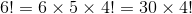# High School Math : Factorials

## Example Questions

### Example Question #1 : Factorials

What is the value of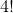?

10

4

6

24

12

24

Explanation:

! is the symbol for factorial, which means the product of the whole numbers less than the given number.

Thus,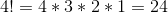### Example Question #1 : Factorials

What is the value of?Explanation:

By definition,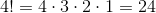### Example Question #3 : Factorials

Find the value of: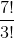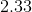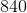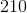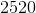Explanation:

The factorial sign (!) just tells us to multiply that number by every integer that leads up to it.  So,can also be written as: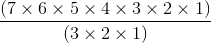To make this easier for ourselves, we can cancel out the numbers that appear on both the top and bottom: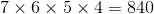### Example Question #4 : Factorials

Solve the following expression.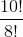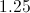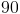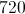Explanation: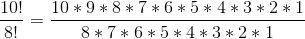This expression can be simplified because all terms in the expression for 8! are also found in the expression for 10!. By writing the expression below, we are able to cancel 8!.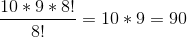### Example Question #1 : Multiplying And Dividing Factorials

Solve: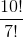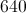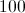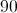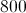Explanation:

Both the numerator and denominator are factorials. If you expanded both, everything would cancel out except for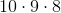in the numerator. Multiply those together to get 720.

### Example Question #6 : Factorials

Simplify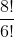.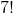Explanation: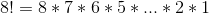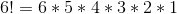Thus,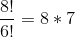since the remaining terms cancel out. 56 is the simplified result.

### Example Question #7 : Factorials

Stewie has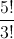marbles in a bag. How many marbles does Stewie have?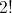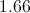Explanation:Simplifying this equation we notice that the 3's, 2's, and 1's cancel so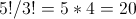Alternative Solution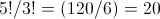### Example Question #8 : Factorials

Which of the following is NOT the same as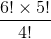?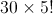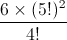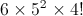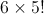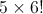Explanation:

Thecancels out all of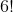except for the parts higher than 4, this leaves a 6 and a 5 left to multilpy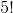### Example Question #9 : Factorials

Simplify and solve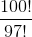.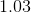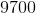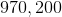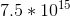Explanation:

Remember a number followed by a ! is a factorial. A factorial is the product of the given number and all of the numbers smaller than it down to zero. For example,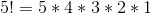.

Rather than do all of the math involved for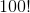, notice thatis the same as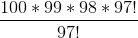From here, the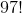's cancel out, leaving us with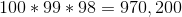### Example Question #10 : Factorials

Which of the following is equivalent to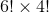?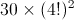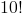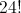None of the other answers are correct.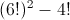This is a factorial question. The formula for factorials is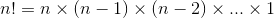.